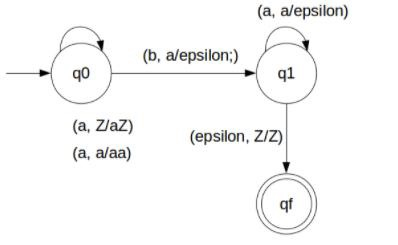# Construct Deterministic PDA for a^n b^n where n>=1

## Problem

Construct deterministic push down automata (DPDA) for anbn where n>=1.

## Solution

So, the strings which are generated by the given language are as follows −

L={ab,aabb,aaabbb,….}

That is we have to count equal number of a’s and b’s

This can be achieved by pushing a's in STACK and then we will pop a's whenever "b" comes.

Finally at the end of the strings if nothing is left in the STACK then we can declare that language is accepted in the PDA.

The transition diagram is as follows −## Transition Functions

The transition functions are as follows −

δ(q0, a, Z) = (q0, aZ)

δ(q0, a, a) = (q0, aa)

δ(q0, b, a) = (q1, ε)

δ(q1, b, a) = (q1, ε)

δ(q1, ε, Z) = (qf, Z)

## Explanation

Step 1 − Let’s take an input string: "aabb".

Step 2 − Scan string from left to right.

Step 3 − First input is 'a' and the rule.

input 'a' and STACK alphabet Z, then

push the input 'a' into STACK : (a,Z/aZ) and state will be q0.

Step 4 − Second input is 'a' and the rule.

input input 'a' and STACK alphabet 'a', then

input push the input 'a' into STACK : (a,a/aa) and state will be q0.

Step 5 − Third input is 'b' and the rule.

input 'b' and STACK alphabet 'a', then

pop STACK with one 'a': (b,a/&epsiloon;) and state will be now q1.

Step 6 − Fourth input is 'b' and the rule.

input 'b' and STACK alphabet 'a' and state is q1, then

pop STACK with one 'a' : (b,a/&epsiloon;) and state will be q1.

Step 7 − We reached the end of the string, the rule.

input ε and STACK alphabet Z, go to final state(qf) : (ε, Z/Z)